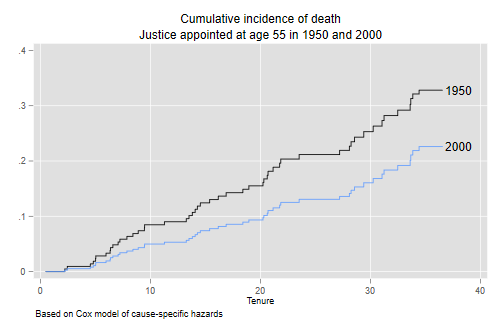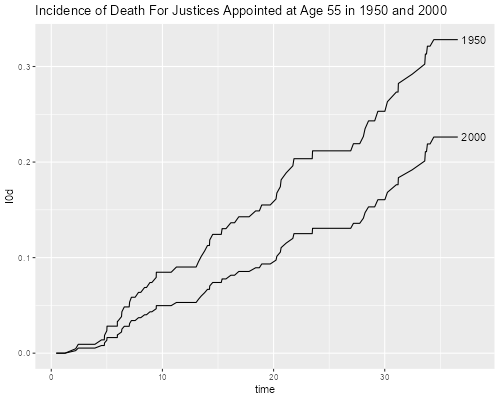Germán Rodríguez
Survival Analysis Princeton University## Cox Models of Competing Risks

We continue our competing risk analysis of supreme court tenure by introducing two covariates: `age`, how old the justice was when appointed, and `year`, the calendar year of appointment. We obviously expect survival to improve over time and decline with the age of the justice at appointment, but it is not clear whether the trend over time is to retire earlier or later.

We use the file created in the previous step, which I have saved on this website.

```. use https://grodri.github.io/datasets/justices2, clear
```
```> justices <- read.csv("https://grodri.github.io/datasets/justices2.csv")
```

We can now run a Cox regression looking at overall tenure in the court

```. stset tenure, fail(event)

Survival-time data settings

Failure event: event!=0 & event<.
Observed time interval: (0, tenure]
Exit on or before: failure

──────────────────────────────────────────────────────────────────────────
113  total observations
0  exclusions
──────────────────────────────────────────────────────────────────────────
113  observations remaining, representing
104  failures in single-record/single-failure data
1,887.365  total analysis time at risk and under observation
At risk from t =         0
Earliest observed entry t =         0
Last observed exit t =  36.57221

. stcox age year, efron

Failure _d: event
Analysis time _t: tenure

Iteration 0:   log likelihood = -389.72292
Iteration 1:   log likelihood = -375.63169
Iteration 2:   log likelihood = -375.56405
Iteration 3:   log likelihood = -375.56403
Refining estimates:
Iteration 0:   log likelihood = -375.56403

Cox regression with Efron method for ties

No. of subjects =        113                            Number of obs =    113
No. of failures =        104
Time at risk    = 1,887.3648
LR chi2(2)    =  28.32
Log likelihood = -375.56403                             Prob > chi2   = 0.0000

─────────────┬────────────────────────────────────────────────────────────────
_t │ Haz. ratio   Std. err.      z    P>|z|     [95% conf. interval]
─────────────┼────────────────────────────────────────────────────────────────
age │   1.084647   .0187453     4.70   0.000     1.048522    1.122017
year │   .9941362   .0017051    -3.43   0.001     .9907998    .9974839
─────────────┴────────────────────────────────────────────────────────────────
```
```> library(survival)
> coxb <- coxph(Surv(tenure, event > 0) ~ age + year, data=justices)
> coef(summary(coxb))
coef exp(coef)    se(coef)         z     Pr(>|z|)
age   0.081254650 1.0846471 0.017282397  4.701585 2.581503e-06
year -0.005881029 0.9941362 0.001715201 -3.428770 6.063235e-04
```

We see that the risk of leaving the court at any length of service is 8.5% higher for every year of age at appointment, and just over half a percent lower per calendar year. This, of course, confounds trends in mortality with trends in retirement.

To look at the risk of death we use death as the event indicator, treating everything else as censoring

```. streset, fail(event == 1) // just death
-> stset tenure, failure(event==1)

Survival-time data settings

Failure event: event==1
Observed time interval: (0, tenure]
Exit on or before: failure

──────────────────────────────────────────────────────────────────────────
113  total observations
0  exclusions
──────────────────────────────────────────────────────────────────────────
113  observations remaining, representing
50  failures in single-record/single-failure data
1,887.365  total analysis time at risk and under observation
At risk from t =         0
Earliest observed entry t =         0
Last observed exit t =  36.57221

. stcox age year, efron

Failure _d: event==1
Analysis time _t: tenure

Iteration 0:   log likelihood = -187.36741
Iteration 1:   log likelihood = -176.31427
Iteration 2:   log likelihood = -176.28608
Iteration 3:   log likelihood = -176.28608
Refining estimates:
Iteration 0:   log likelihood = -176.28608

Cox regression with no ties

No. of subjects =        113                            Number of obs =    113
No. of failures =         50
Time at risk    = 1,887.3648
LR chi2(2)    =  22.16
Log likelihood = -176.28608                             Prob > chi2   = 0.0000

─────────────┬────────────────────────────────────────────────────────────────
_t │ Haz. ratio   Std. err.      z    P>|z|     [95% conf. interval]
─────────────┼────────────────────────────────────────────────────────────────
age │   1.070161   .0251142     2.89   0.004     1.022053    1.120533
year │    .988923   .0025736    -4.28   0.000     .9838917    .9939801
─────────────┴────────────────────────────────────────────────────────────────
```
```> coxd <- coxph(Surv(tenure, event == 1) ~ age + year, data=justices)
> coef(summary(coxd))
coef exp(coef)    se(coef)         z     Pr(>|z|)
age   0.06780911  1.070161 0.023467640  2.889473 3.858885e-03
year -0.01113878  0.988923 0.002602433 -4.280141 1.867747e-05
```

We see that the risk of death while in the court is about 7% higher per year of age at appointment, and declines just over one percent per calendar year of appointment, reflecting general improvements in mortality over time. Note that using dates in days we had no ties.

To look at the risk of retirement we change the event of interest

```. streset , fail(event == 2) // just retirement
-> stset tenure, failure(event==2)

Survival-time data settings

Failure event: event==2
Observed time interval: (0, tenure]
Exit on or before: failure

──────────────────────────────────────────────────────────────────────────
113  total observations
0  exclusions
──────────────────────────────────────────────────────────────────────────
113  observations remaining, representing
54  failures in single-record/single-failure data
1,887.365  total analysis time at risk and under observation
At risk from t =         0
Earliest observed entry t =         0
Last observed exit t =  36.57221

. stcox age year, efron

Failure _d: event==2
Analysis time _t: tenure

Iteration 0:   log likelihood = -202.36566
Iteration 1:   log likelihood = -193.54943
Iteration 2:   log likelihood = -193.28902
Iteration 3:   log likelihood = -193.28867
Refining estimates:
Iteration 0:   log likelihood = -193.28867

Cox regression with no ties

No. of subjects =        113                            Number of obs =    113
No. of failures =         54
Time at risk    = 1,887.3648
LR chi2(2)    =  18.15
Log likelihood = -193.28867                             Prob > chi2   = 0.0001

─────────────┬────────────────────────────────────────────────────────────────
_t │ Haz. ratio   Std. err.      z    P>|z|     [95% conf. interval]
─────────────┼────────────────────────────────────────────────────────────────
age │   1.105598   .0281334     3.95   0.000     1.051811    1.162137
year │    .999077   .0024063    -0.38   0.701     .9943719    1.003804
─────────────┴────────────────────────────────────────────────────────────────
```
```> coxr <- coxph(Surv(tenure, event == 2) ~ age + year, data=justices)
> coef(summary(coxr))
coef exp(coef)    se(coef)          z     Pr(>|z|)
age   0.1003868036  1.105598 0.025446280  3.9450482 7.978392e-05
year -0.0009234354  0.999077 0.002408505 -0.3834061 7.014186e-01
```

We see an increase in the risk of retirement of 10.6% per year of age at appointment, and essentially no trend over time, so the risk of retirement depends only on starting age and not on calendar year of appointment.

### Incidence from Cox Models

The next step will be to calculate cumulative incidence functions based on the Cox models for cause-specific hazards. In the next section we will use the Fine and Gray model.

The aim is to compare the cumulative incidence of death for justices appointed at age 55 in 1950 and 2000. For convenience I will center age on 55 and calendar year on 1950. In both cases I add 0.5 to convert to exact age and the middle of the year. We start by fitting a Cox model to the risk of death

```. gen agec = age - 55.5

. gen yearc = year - 1950.5

. streset, fail(event == 1)
-> stset tenure, failure(event==1)

Survival-time data settings

Failure event: event==1
Observed time interval: (0, tenure]
Exit on or before: failure

──────────────────────────────────────────────────────────────────────────
113  total observations
0  exclusions
──────────────────────────────────────────────────────────────────────────
113  observations remaining, representing
50  failures in single-record/single-failure data
1,887.365  total analysis time at risk and under observation
At risk from t =         0
Earliest observed entry t =         0
Last observed exit t =  36.57221

. quietly stcox agec yearc
```
```> library(dplyr)
> justices <- mutate(justices,
+     agec = age - 55.5,
+     yearc = year - 1950.5
+ )
> coxd <- coxph(Surv(tenure, event == 1) ~ agec + yearc, data=justices)
```

The next task is to calculate the baseline hazard. I will do this by calculating the Nelson-Aalen estimate of the cumulative hazard and then differencing it. I think this is conceptually clearer, and yields results that are very similar to an alternative calculation based on the Kalbfleisch-Prentice estimate of the survival function. I also calculate the hazard for a judge appointed 50 years later using the estimated coefficient from the Cox regression.

```. predict H0d, basech

. gsort +_t -_d

. gen h0d = H0d - H0d[_n - 1]
(1 missing value generated)

. replace h0d = H0d in 1

. gen h1d = h0d*exp(50 * _b[yearc])
```
```> sfd <- survfit(coxd, newdata=list(agec = 0, yearc = 0))
> cif <- data.frame(time = sfd\$time,
+     h0d = diff(c(0, sfd\$cumhaz)))
> cif <- mutate(cif, h1d = h0d * exp(50 * coef(coxd)["yearc"]))
```

The next step is to do exactly the same calculations for the risk of retirement

```. streset, fail(event == 2)
-> stset tenure, failure(event==2)

Survival-time data settings

Failure event: event==2
Observed time interval: (0, tenure]
Exit on or before: failure

──────────────────────────────────────────────────────────────────────────
113  total observations
0  exclusions
──────────────────────────────────────────────────────────────────────────
113  observations remaining, representing
54  failures in single-record/single-failure data
1,887.365  total analysis time at risk and under observation
At risk from t =         0
Earliest observed entry t =         0
Last observed exit t =  36.57221

. quietly stcox agec yearc

. predict H0r, basech

. gsort +_t -_d

. gen h0r = H0r - H0r[_n - 1]
(1 missing value generated)

. replace h0r = H0r in 1

. gen h1r = h0r * exp(50 * _b[yearc])
```
```> coxr <- coxph(Surv(tenure, event == 2) ~ agec + yearc, data=justices)
> sfr <- survfit(coxr, newdata=list(agec = 0, yearc = 0))
> if(!all(sfr\$time == sfd\$time)) stop("times differ")
> cif <- mutate(cif,
+     h0r = diff(c(0, sfr\$cumhaz)),
+     h1r = h0r * exp(50 * coef(coxr)["yearc"])
+ )
```

We can now add the hazards of death and retirement and calculate the overall survival probability as well as the incidence functions, all from first principles. For these calculations we need to retain just one observation per distinct failure time and make sure the hazards for all causes are attached to the same observation, which we do by taking the maximum for each value of `_t`. Hat tip to Shawna Metzger, who alerted me to this issue and provided the solution. In this dataset there is only one tie between a death and a retirement at 1832 days or 5.0157 years. In the original dataset there were many ties, as dates were coded in years only.

```. foreach hazard in h0d h0r h1d h1r {
2.     capture drop temp
3.     egen temp = max(`hazard'), by(_t)
4.     quietly replace `hazard' = temp
5. }

. egen first = tag(_t)

. drop if !first
(1 observation deleted)
```

We then calculate the incidence functions of death for 1950 and 2000, summing the product of the probabilities of surviving all causes of attrition up to each time and then failing due to each cause:

```. forvalues y = 0/1 {
2.     gen h`y' = h`y'd + h`y'r
3.     gen S`y' = exp(-sum(h`y')) // Aalen
4.     gen c`y' = S`y'[_n-1] * h`y'd
5.     replace c`y' = h`y'd in 1
6.     gen I`y' = sum(c`y')
7. }
(1 missing value generated)
(1 missing value generated)

. line I0 I1 _t, connect(J J) ///
>     title("Cumulative incidence of death") xtitle(Tenure) ///
>     subtitle("Justice appointed at age 55 in 1950 and 2000") ///
>     note(Based on Cox model of cause-specific hazards) ///
>     text(.226 38 "2000") text(.328 38 "1950") legend(off)

. graph export justices-cifcox.png, width(500) replace
file justices-cifcox.png saved as PNG format

. list I0 I1 in -1

┌─────────────────────┐
│       I0         I1 │
├─────────────────────┤
112. │ .3281087   .2261896 │
└─────────────────────┘
``````> n <- nrow(cif)
> cif <- mutate(cif,
+     S0 = exp(-cumsum(h0d + h0r)),
+     S1 = exp(-cumsum(h1d + h1r)),
+     I0d = cumsum(c(1, S0[-n]) * h0d),
+     I1d = cumsum(c(1, S1[-n]) * h1d)
+ )
> cif[n, c("time", "I0d", "I1d")]
time       I0d       I1d
112 36.57221 0.3281087 0.2261896
> library(ggplot2)
> g <- ggplot(cif, aes(time, I0d)) + geom_line() + geom_line(aes(time, I1d)) +
+ ggtitle("Incidence of Death For Justices Appointed at Age 55 in 1950 and 2000")
> g + annotate("text", x = 38, y=max(cif\$I0d), label="1950") +
+     annotate("text", x = 38, y=max(cif\$I1d), label="2000")
> ggsave("justices-cifcoxr.png", width = 500/72, height = 400/72, dpi = 72)
```We see that the probability of dying while serving in the supreme court for a judge appointed at age 55 has fallen from 32.8% in 1950 to 22.6% in 2000, assuming of course that the trends continue so the model still applies.

Note. You may be interested to know that using an approach analogous to the Kaplan-Meier estimate yields the same probabilities quoted above to three decimal places.

